PetroSkills Blog
By:

# Part 2 – Thermodynamic Hydrate Inhibitors Series

Currently, there are several different thermodynamic hydrate inhibitors that one has available to choose from.  One could choose to use an oxygenate, such as methanol, or a glycol, such as mono-ethylene glycol (MEG) or diethylene glycol (DEG).  But what determines the inhibitors hydrate suppression performance, and which one should I choose for my inhibition needs? The primary considerations for an application include:

1. Hydrate Suppression Effectiveness

2. Inhibitor Regeneration Requirements

3. Inhibitor Losses / Product Contamination

4. Inhibitor Cost

Hydrate Suppression Effectiveness

The lower the molecular weight of a thermodynamic inhibitor, the better the hydrate suppression performance.  For example, in glycols MEG has better performance characteristics than DEG, and methanol MeOH will outperform ethanol EtOH. In Part 1 of this tip of the month (November 2020 TOTM ) provided insight into the relative performance of hydrate inhibitors, and discussed the limitations, pros and cons of the various options.  Part 1 demonstrated that for a hydrate depression temperature of 10 oC (18oF) to prevent hydrates formation in our gathering system the required concentration of inhibitor in the liquid water phase using the Nielsen and Bucklin  equation is 13 mole %.  Figure 1 provides the corresponding weight % of the various inhibitors that would be required for this depression temperature (d). As shown in Figure 1, the inhibitors weight % is a function of molecular weight shown on the horizontal axes.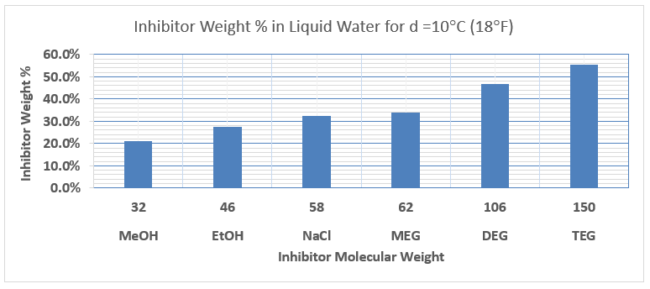Figure 1. Comparison of Inhibitor Requirements in Liquid Water Phase for Depression Temperature of 10oC (18oF)

In this TOTM we will review a Case Study in the application and selection of inhibitor for a gathering system inhibition application. Read Part One here.

Case Study

To prepare a hydrate prevention program for the subsea pipeline, let us consider a multiphase subsea pipeline transporting gas and condensate from an offshore production facility to shore. The flow rates and pipeline conditions are provided in Table 1. The feed to the pipeline is free of liquid water and the condensate has no toluene. The main objective is to estimate hydrate formation temperature expected in the pipeline and make a recommendation on type of thermodynamic inhibitor to be used.  For the methanol injection estimates, we will include both the vapor and liquid hydrocarbon losses.

Table 1. Flow rates and pipeline conditions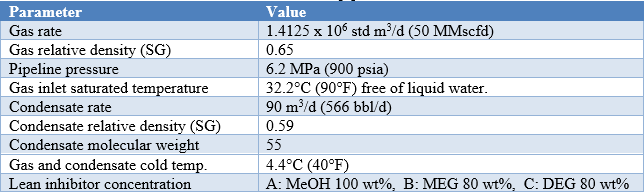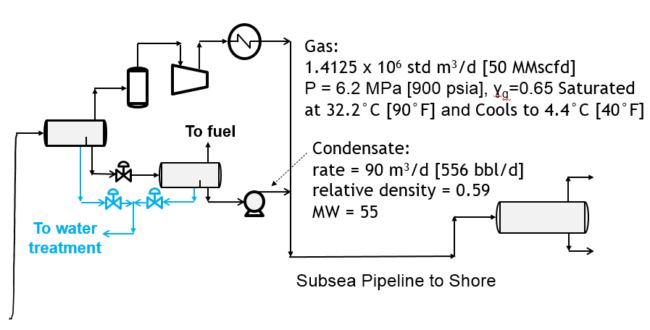Figure 2. Schematic of subsea pipeline

The water content of lean sweet natural gas is a function of temperature and pressure and can be estimated from the charts in chapter 6 of volume 1 of Gas Conditioning and Processing . The water content of the gas at the inlet and outlet of pipeline are used to the determine the amount of condensed water in the pipeline. The condensed water is a first key factor that establishes the amount of hydrate inhibitor required. Likewise, the hydrate formation temperature (HFT) is a function of pressure and relative density of gas and can be estimated from the chart in reference . The second key factor is the required depression temperature (d=HFT-Tcold).  The HFT is an important parameter for determination of the depression temperature. The summary of preliminary calculation results is presented in Table 2.

Table 2. Summary of preliminary calculation resultsThe hydrate inhibitor performance plots that are presented in this tip were developed using the Nielsen and Bucklin  correlation presented as equation 1.The third key factor is the required inhibitor weight % in the liquid water phase, XR.  From Figure 3 for depression temperature. d = 12.1 °C (22 °F), XR for MeOH, MEG and DEG are determined and shown in Table 3. The inhibitor concentration in the same phase for all three inhibitors is xR 15.6 mole %.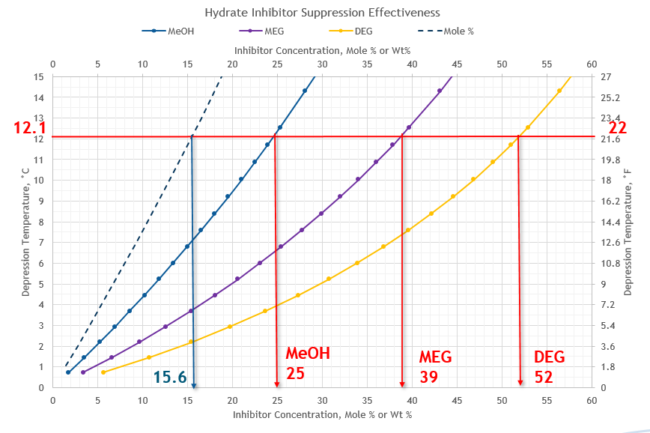Figure 3.  Depression temperature vs mole % and weight % hydrate inhibitor in the liquid water phase for Typical Gathering Systems

From Figure 4 for depression temperature, d = 12.1 °C (22 °F), the required ratio of mass of inhibitor in the liquid water phase to mass of condensed water, (m/ mW) for three inhibitor are determined and shown in Table 3. The required inhibitor rate in liquid water, mI, the fourth key factor, can be calculated by equation 2.From Figure 4 at the same depression temperature, the required mass ratio, (m/ mW), for lean MEG 100 wt% and DEG 100 wt%, are 0.63% and 1.08, respectively.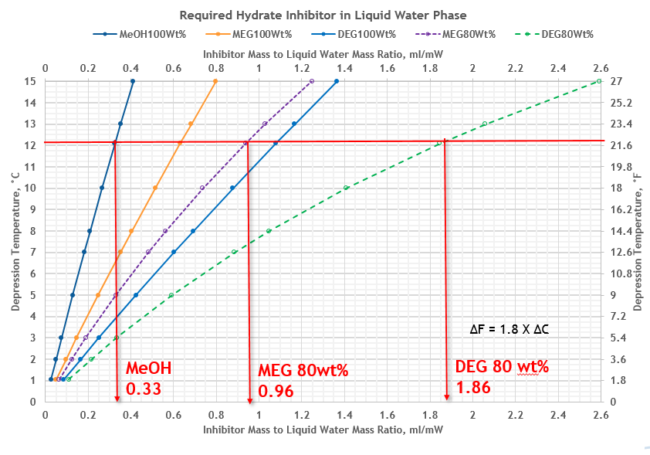Figure 4.  Depression temperature vs ratio of mass of inhibitor to mass of condensed water, mI/mW

The summary of the required inhibitors rate calculations for liquid water phase are presented in Table 3.

Table 3. Summary of required inhibitors injection rate for liquid water phase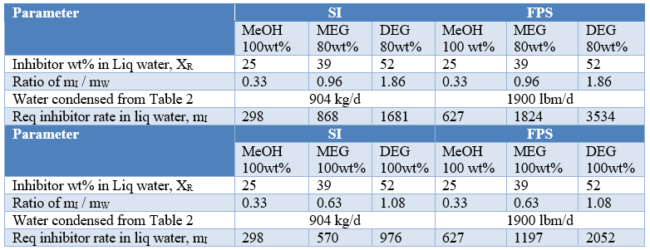Figure 5 and Table 3 present comparison of inhibitors-to-condensed water mass ratio, (m/ mW), requirements for MeOH, MEG and DEG at various lean inhibitor weight %. Figure 5 indicates that methanol with MW=32 is the most effective because of its lowest mass ratio requiring the lowest inhibitor mass rate while DEG with MW = 106 is the least effective because its largest mass ratio requiring the most inhibitor mass rate.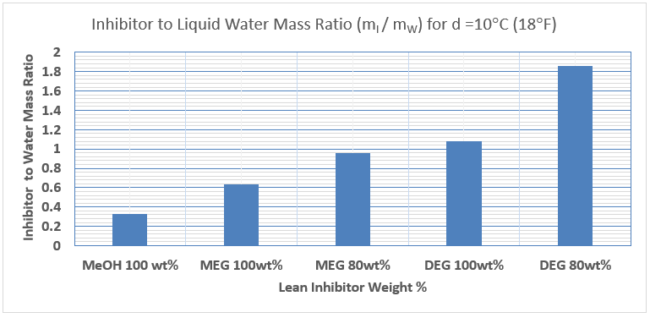Figure 5.  Comparison of inhibitors to liquid water mass ratio, (m/ mW)

MeOH Losses to Liquid and Vapor Hydrocarbon Phase

Due to low vapor pressure and low solubility in liquid hydrocarbons, glycol losses to vapor and liquid hydrocarbon phases are negligible, and we will assume these to be zero. However, MeOH losses to vapor and liquid hydrocarbon phases are significant and estimated in this tip.

The MeOH losses to liquid hydrocarbon phase can be estimated by our proposed Figure 6 and equation 3.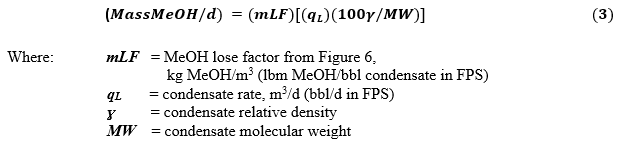From Figure 6 for depression temperature, d = 12.1 °C (22 °F), the required MeOH loss factor is 0.44 kg/m3 (0.154 lbm/bbl). For a condensate rate of 90 m3/d (566 bbl/d), relative density of ɣ = 0.59 and molecular weight of MW = 55, calculate rate of MeOH loss to liquid hydrocarbon phase by equation 3.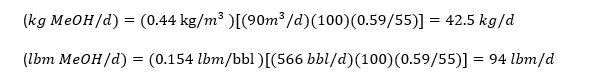Figure 6. MeOH loss factor to liquid hydrocarbon phase

The MeOH losses to vapor hydrocarbon phase can be estimated by our proposed equation 4 and Figure 7 .From Figure 7 for cold temperature of 4.4 °C (40 °F) and pressure of 6.2 MPa (900 psia) the K value is 0.0019. For agas rate of 1.4125 x 106 std m3/d (50 MMscfd) and MeOH mole fraction of x=0.156, calculate rate of MeOH losses to vapor hydrocarbon phase by equation 4.Figure 7. MeOH K-value in natural gas

The total required inhibitors injection rate to prevent hydrate formation is calculated by equation 5.Table 4 presents summary of total required inhibitors injection rate to prevent hydrate formation. Figure 8 also presents comparison of water phase inhibitor rate compared (blue bars) to total required inhibitors injection rate (red bars) to prevent hydrate formation.

Table 4. Summary of total required inhibitors injection rate to prevent hydrate formation.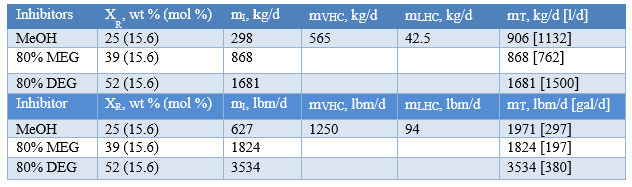Figure 8. Comparison of total required inhibitors injection rate to prevent hydrate formation.

Despite MeOH excellent performance in terms of hydrate suppression, its biggest downfall is its distribution behavior.  As shown in Table 4 and Figure 8, methanol was distributed in all three phases of liquid water, liquid and vapor hydrocarbon phases. In fact, the losses are significantly greater than the rate required in the water phase which results in higher injection rates required as compared to MEG and DEG. For our Case Study the sum of MeOH losses to the vapor and liquid hydrocarbon phases is two times greater than the required rate of inhibitor in the liquid water phase. The amount of MeOH loss to the vapor phase is a function of pressure, temperature, and mole fraction of MeOH in the liquid water phase. But the amount of MeOH loss to the condensate phase is a function of temperature, mole fraction of MeOH in the liquid water phase condensate relative density and molecular weight. Note that mole fraction of MeOH in the liquid water phase is a function of depression temperature.

Once you inject methanol into the pipeline, it is in the gas, the condensed water, and the liquid hydrocarbons.  This is becoming a more significant issue these days.  Too much MeOH in the produced water can kill the microbes in the produced water treatment system (and it is toxic to fish so produced water offshore could not go overboard). In addition, MeOH is a petrochemical catalyst poison.  Many North American natural gas, y-grade (NGL), and liquid products pipeline tariffs are specifying a maximum oxygenate concentration – or specifying ZERO!.   In addition, methanol will slowly kill your very expensive 4A molecular sieve dehydration bed over time.

Glycols on the other hand have very low vapor pressure, with minimal losses in the vapor phase.  In addition, there is very little mutual solubility with liquid hydrocarbons, so the glycol STAYs where you want it to be, in the water phase.

Inhibitor Cost

Methanol is cheap in comparison to glycols.  As a rough rule of thumb, glycols are roughly three times the cost of methanol.   That is why glycols are nearly always regenerated.  You only need to invest in the chemical costs once.  However, methanol is cheap, but you will need to continually buy the amount of chemical you are injecting as it goes down stream and is not recovered.

Summary

This TOTM presented two new charts, Figures 4 and 6, and their corresponding equations. Both figures and equations are simple and easy to use. Figure 4 can be used to estimate the required inhibitor injection rate in liquid water phase for specified depression temperature and rate of water condensed.  Figure 6 can be used to estimate the rate of MeOH loss to the liquid hydrocarbon phase if depression temperature, cold temperature and condensate relative density are known. This TOTM also reviewed a Case Study in the application and selection of inhibitor for a gathering system inhibition application.   In addition, the pro’s and con’s of oxygenates versus glycols was discussed in more detail.

To learn more about similar cases and how to minimize operational problems, we suggest attending our G4 (Gas Conditioning and Processing), G5 (Practical Computer Simulationand Applications in Gas Processing) courses.

By: Kindra Snow-McGregor, P.E., and Mahmood Moshfeghian, Ph.D.

## Sidebar

### Featured posts###### Hydrogen Series Part 1: Hydrogen Colors – What do they mean, Options for Production and their associated Challenges?
by Kindra Snow-McGregor

### Popular tags

#Renewables #LNG #Unconventionals